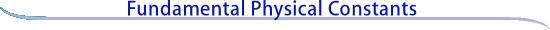Constants Topics: Values Energy Equivalents Searchable Bibliography Background Constants Bibliography Constants, Units & Uncertainty home pageIntroduction to the constants for nonexperts

 Introduction 1900 - 1920 1920 - 1940 1940 - 1960 Current (index)

The fundamental-constants field has advanced so rapidly since mid 20th century that nearly all of the measurements carried out before World War II may be considered historical (if not the method, at least the result). Indeed, few measurements of constants existed before about the turn of the 20th century, because not until then did the modern era of physics begin. Relativity, atomic physics, and quantum theory all emerged after 1900. Two of the more important historical measurements made before about 1920 are:

 The elementary charge (e)

One of the earlier experiments to measure a fundamental constant to high accuracy, as well as an example of how the accurate determination of a fundamental constant using different methods can lead to an improved understanding of a particular physical phenomenon, was the measurement of the fundamental unit of charge (e) by Robert A. Millikan, a physicist in the United States. From about 1907 to 1917 he carried out his now-famous oil drop experiment to determine e. In this method, the displacement of small, charged oil drops (the charge on the drop is usually just a few e) moving in air between two horizontal and parallel metal plates (with and without an applied known voltage) is followed as a function of time. The value of the fundamental constant e is then calculated from many observations on different drops and knowledge of other relevant quantities, especially the viscosity (resistance to flow) of the air. Millikan's final value, reported in 1917, was: (4.774 ± 0.002) x 10-10 esu (esu being the electrostatic unit, one of the units of charge in the centimeter-gram-second [cgs] system of units; this cgs-esu system was in wide use before the general adoption of the SI system).

That this value was significantly in error became clear in the 1930s with the development of a new but indirect method for obtaining the value of e. The technique consisted of separately measuring N, the Avogadro constant (the number of atoms or molecules contained in a mole, which is defined as a mass in grams equal to the atomic or molecular weight of a substance), and F, the Faraday constant (the amount of charge that must pass through a solution to electrolytically deposit a mole of a singly charged, or monovalent element contained in the solution). These two quantities are related by the simple equation that states that the Faraday constant is equal to the Avogadro constant times unit of charge, or F = Ne. It therefore follows that e = F/N, so that the constant e can readily be obtained if the two constants, Faraday and Avogadro, are known.

The Avogadro constant (N) was determined by measuring the density, molecular weight, and crystal lattice spacing of a particular crystal species such as rock salt, using x-ray techniques. The Faraday (F) was determined by measuring the mass of material (e.g., silver) electrolytically deposited onto an electrode when a known current flowing for a known time was allowed to pass through a solution containing the material. The indirect value of the elementary charge (e) deduced in this way was (4.8021 ± 0.0009) x 10-10 esu, significantly different from the Millikan value. The major source of this disturbing discrepancy was traced in the latter part of the 1930s to the use by Millikan of an incorrect value for the viscosity of air. Millikan had taken a value that was almost entirely based on a measurement by one of his students; but it was later shown that the student had made a rather subtle experimental error. When Millikan's data were reevaluated with a correctly determined value for the viscosity of air, the value of e obtained agreed with the indirect value calculated from the Faraday and the Avogadro constant.

Although this case is an example of the general fact that the experimentally determined value of a constant varies with each determination, it must be realized that it is just these variations from determination to determination in the measured numerical values of the constants that often furnish important clues to errors in experiment and theory.

 Ratio of Planck's constant (h) to the elementary charge (e), h/e

The very first precision determination of the ratio h/e used the photoelectric effect: when light of a particular wavelength is allowed to impinge upon a metal surface, electrons are emitted from the surface. If a retarding voltage, or potential, is applied to the metal so that the electrons are just prevented from leaving the surface, then a unique relationship can be shown to exist between the wavelength of the light, the voltage, and the ratio h/e. Millikan, using sodium and lithium, first reported a result from this method in 1916.

A second method to determine the h/e ratio is the so-called short wavelength limit of the continuous x-ray spectrum. In this technique, a beam of electrons is accelerated through a known voltage and is allowed to strike a metal target. The maximum-energy x-ray (that is, the one having the highest frequency or shortest wavelength) is emitted when all of the electrical potential energy of an electron in the beam is converted to a single x-ray photon. By measuring the voltage and the wavelength of the emitted x-ray, the ratio h/e can be determined. The first precision measurement of this type was reported in 1921.

Continue to 1920-1940

Reproduced with permission from Encyclopaedia Britannica, 15th edition. © 1974 Encyclopaedia Britannica, Inc.